# RD Sharma Solutions Class 9 Maths Chapter 23 Graphical Representation Of Statistical Data Exercise 23.1

Class 9 Chapter 23 – Graphical Representation of Statistical Data Exercise 23.1 solutions are provided here. Graphs help in analyzing the time and frequency distribution by providing a clear account and precise picture of any problem. Graphical Representation of Statistical Data is easy to understand and analyze big data or information. RD Sharma Solutions Class 9 Chapter 23  are outlined and solved by the experts at BYJU’S.

## Download PDF of RD Sharma Solutions for Class 9 Maths Chapter 23 Graphical Representation of Statistical Data Exercise 23.1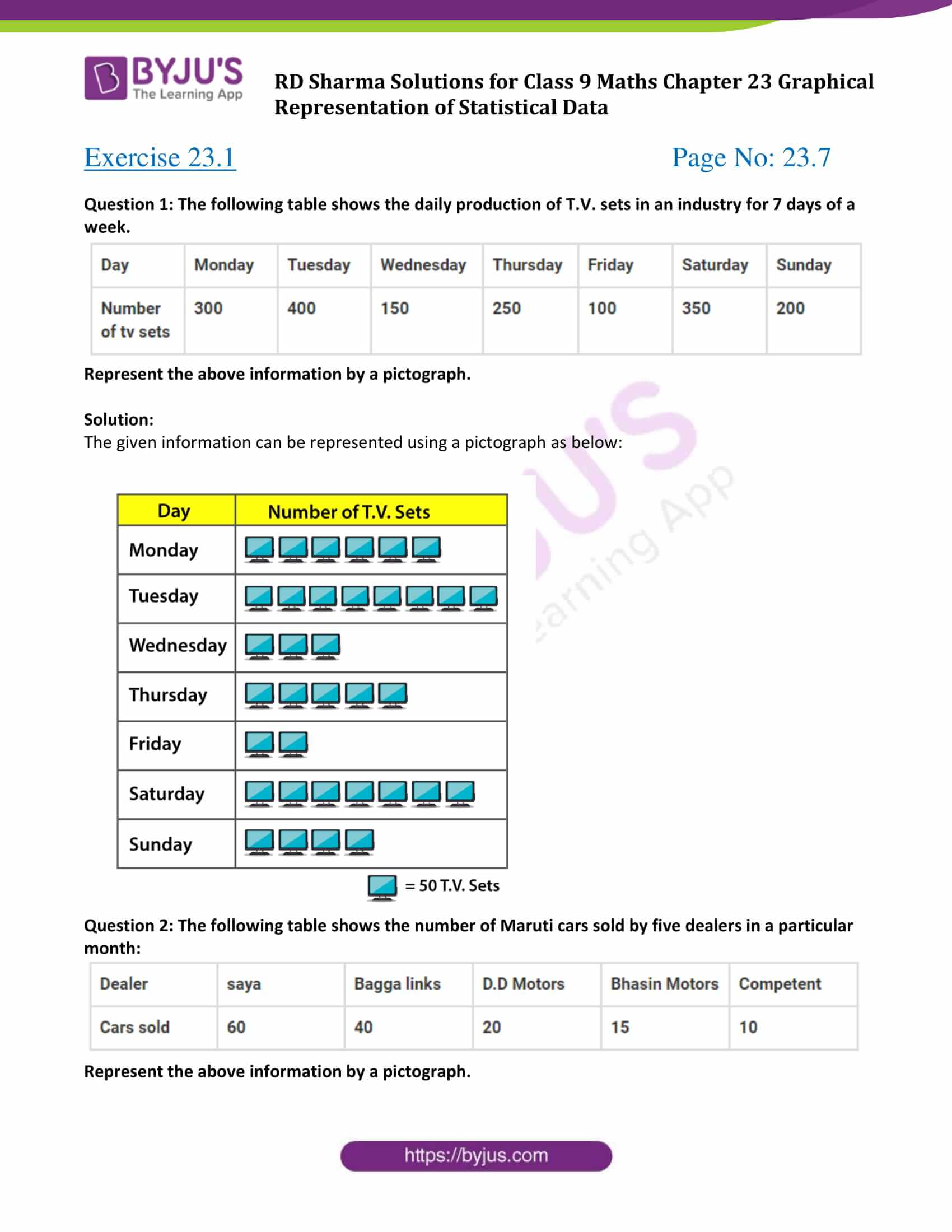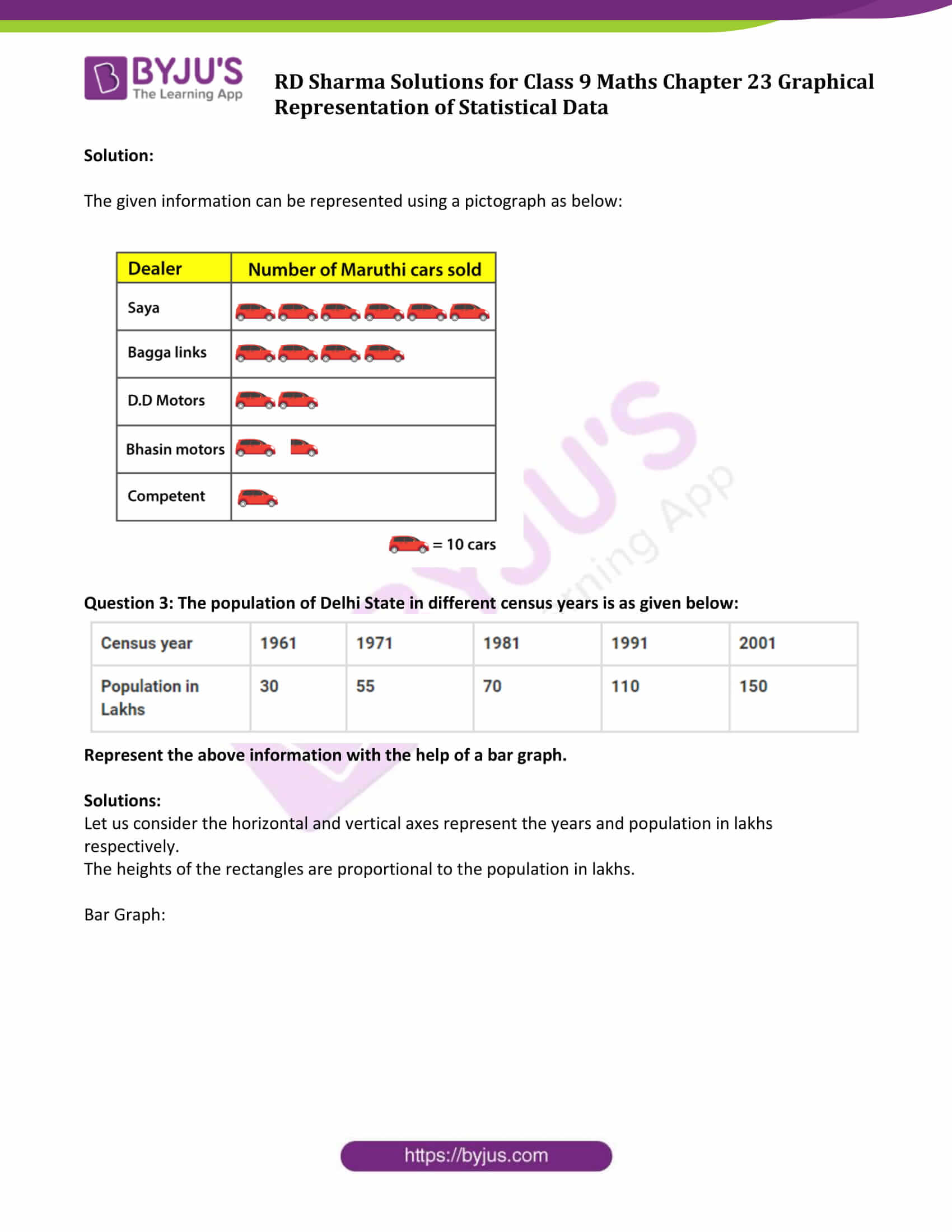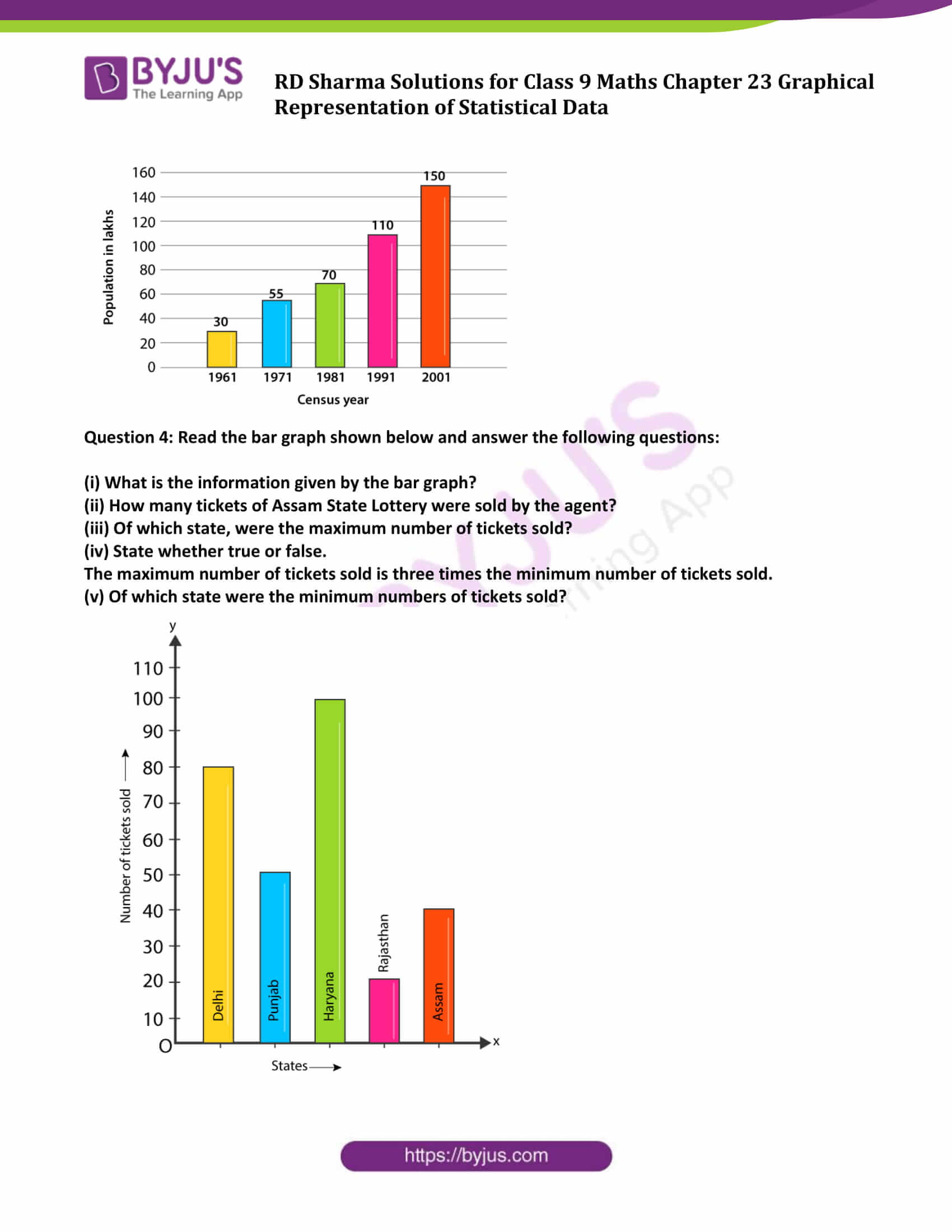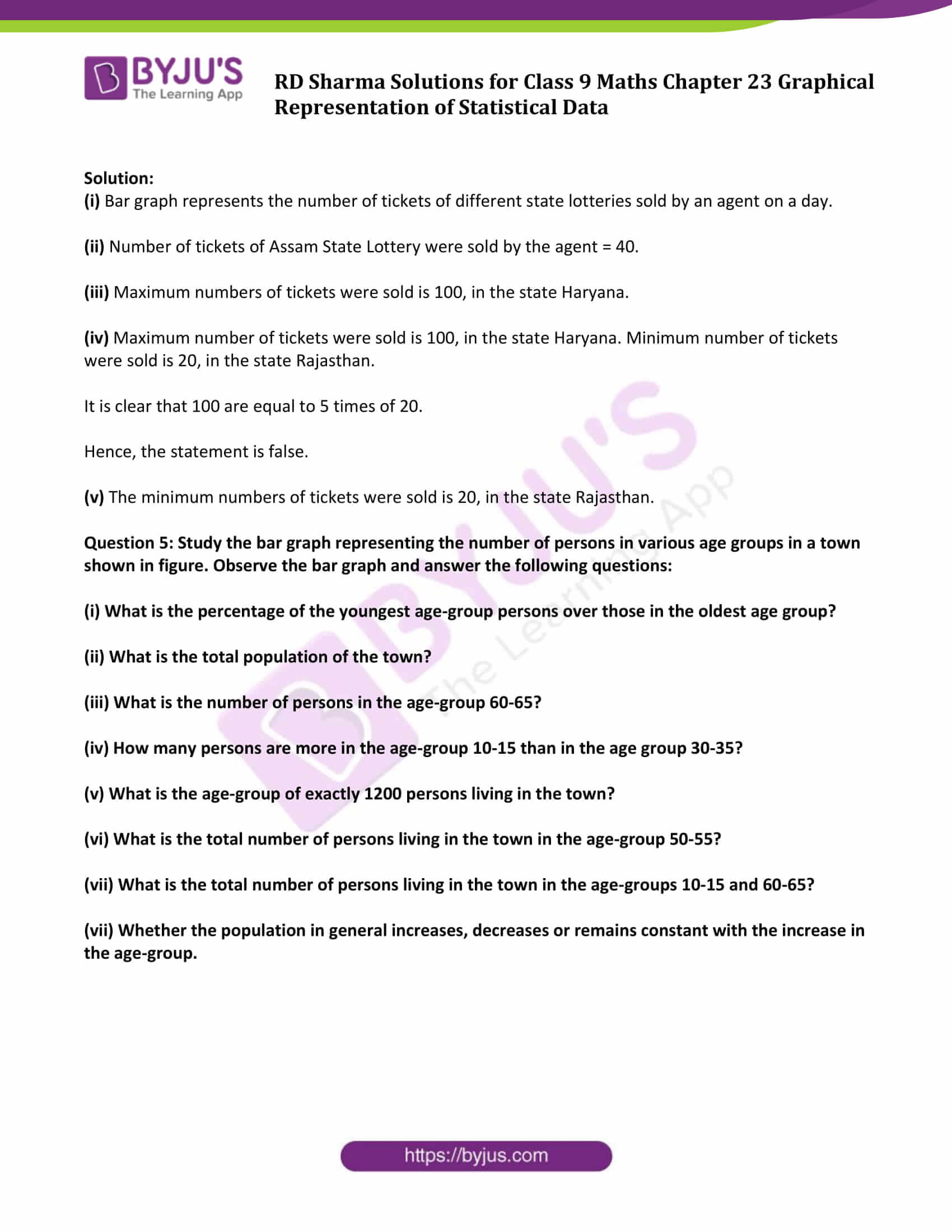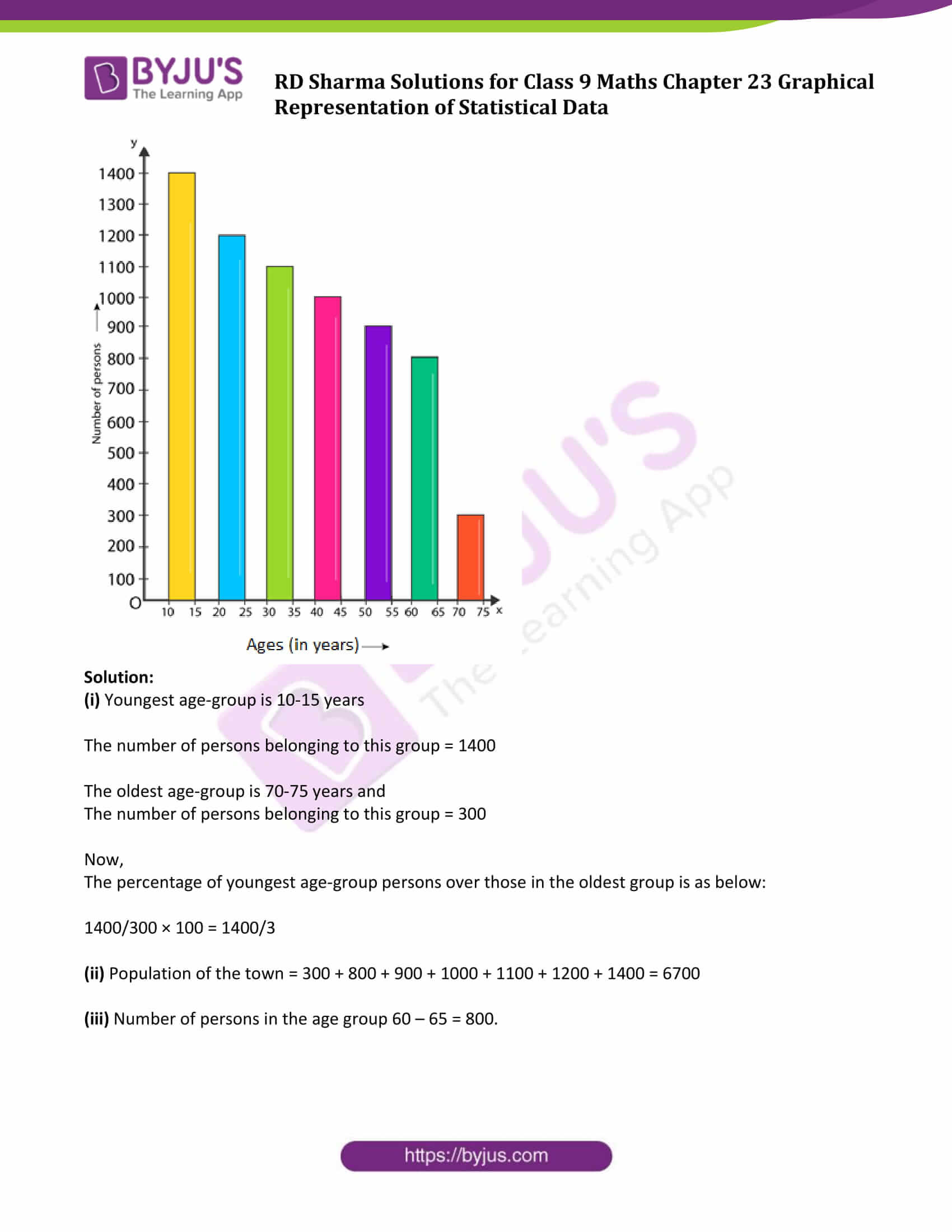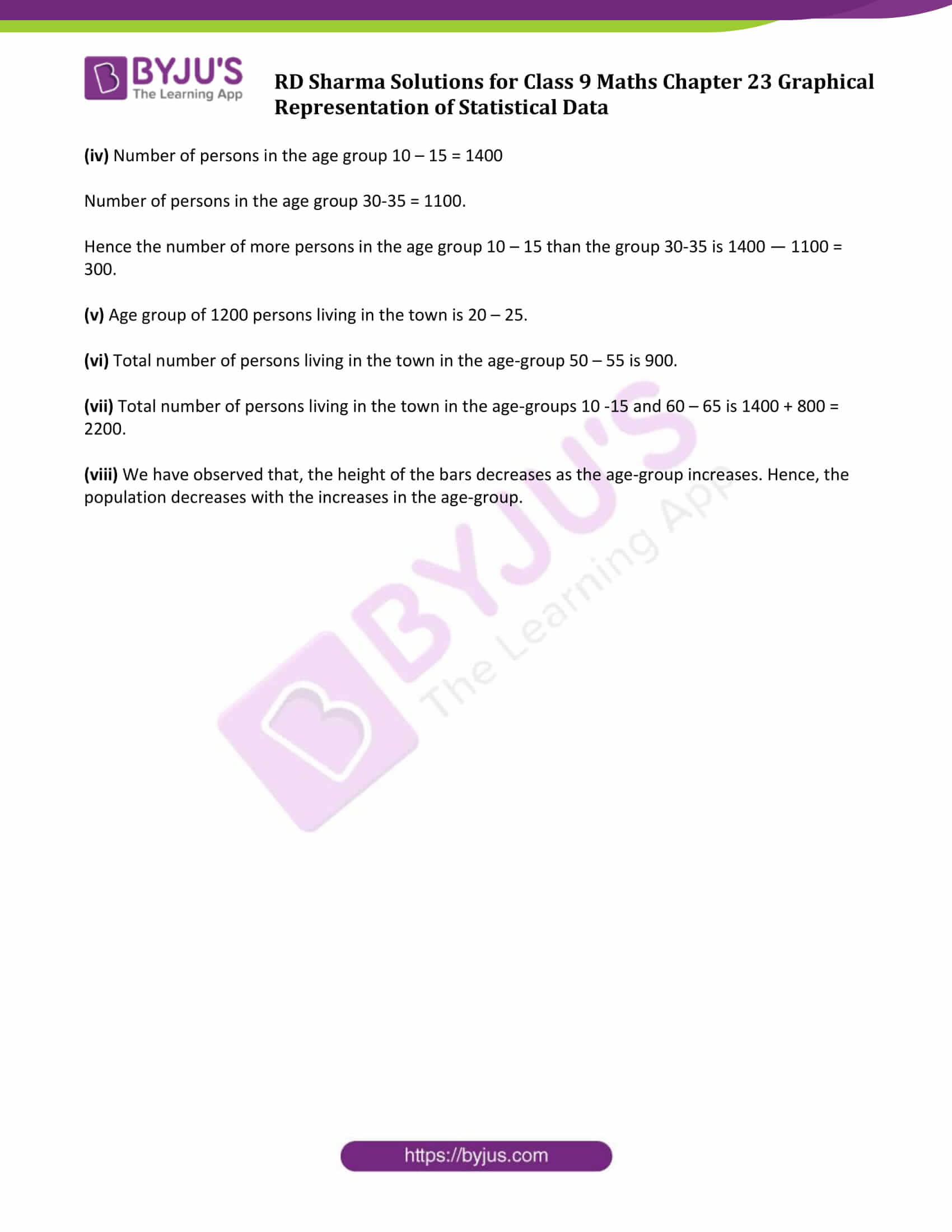### Access Answers to Maths RD Sharma Solutions for Class 9 Chapter 23 Graphical Representation of Statistical Data Exercise 23.1 Page number 23.7

Question 1: The following table shows the daily production of T.V. sets in an industry for 7 days of a week.

 Day Monday Tuesday Wednesday Thursday Friday Saturday Sunday Number of tv sets 300 400 150 250 100 350 200

Represent the above information by a pictograph.

Solution:

The given information can be represented using a pictograph as below: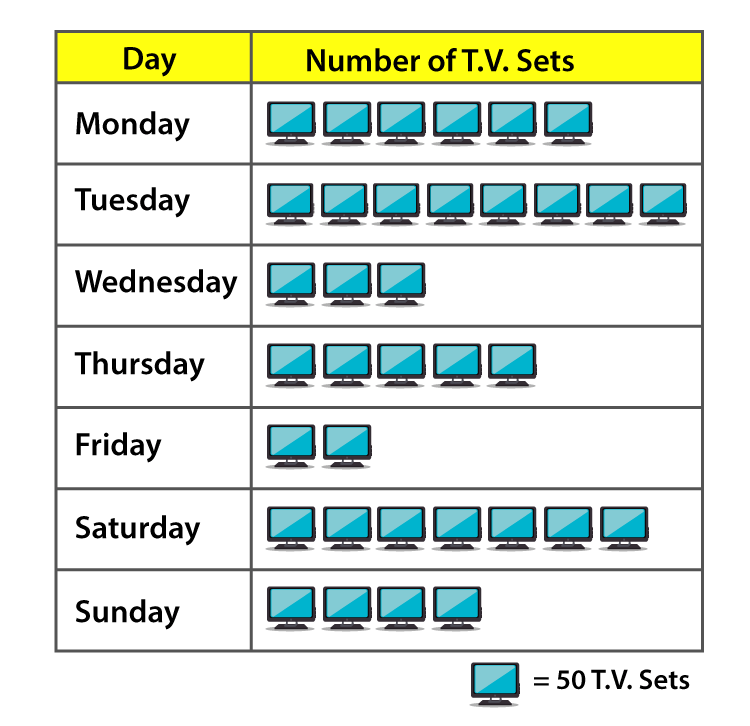Question 2: The following table shows the number of Maruti cars sold by five dealers in a particular month:

 Dealer saya Bagaa links DD motors Bhasin Motors competent cars sold 60 40 20 15 10

Represent the above information by a pictograph.

Solution:

The given information can be represented using a pictograph as below: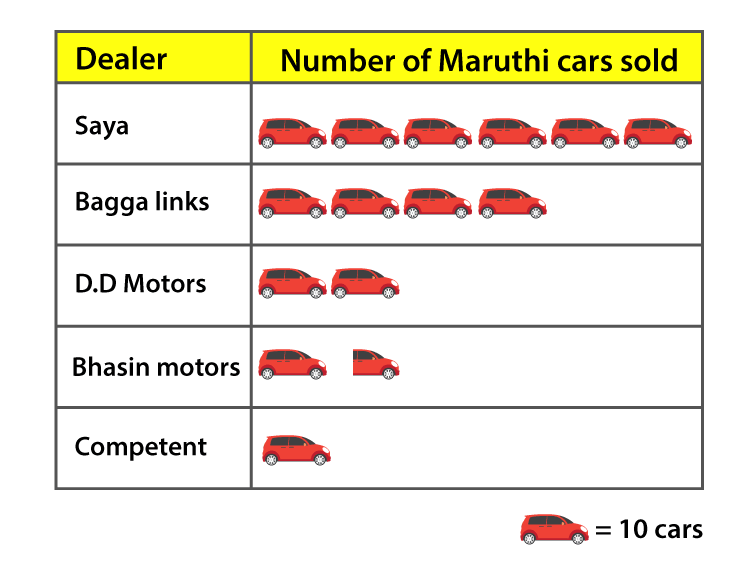Question 3: The population of Delhi State in different census years is as given below:

 Cenus year 1961 1971 1981 1991 2001 Population in lakhs 30 55 70 110 150

Represent the above information with the help of a bar graph.

Solutions:

Let us consider the horizontal and vertical axes represent the years and population in lakhs respectively.

The heights of the rectangles are proportional to the population in lakhs.

Bar Graph: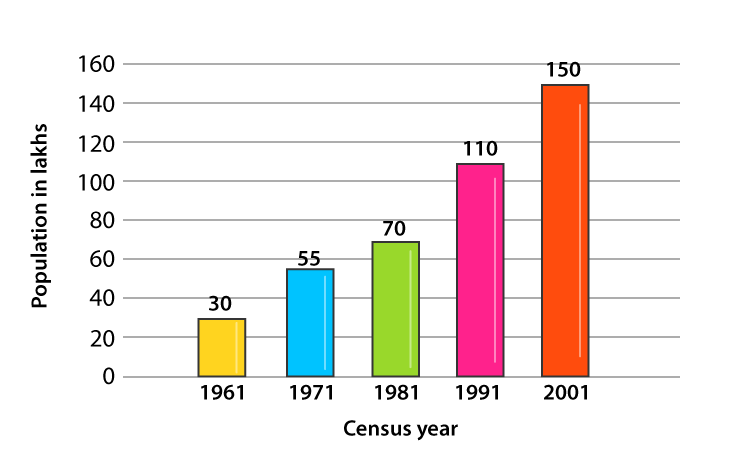Question 4: Read the bar graph shown below and answer the following questions:

(i) What is the information given by the bar graph?

(ii) How many tickets of Assam State Lottery were sold by the agent?

(iii) Of which state, were the maximum number of tickets sold?

(iv) State whether true or false.

The maximum number of tickets sold is three times the minimum number of tickets sold.

(v) Of which state were the minimum numbers of tickets sold?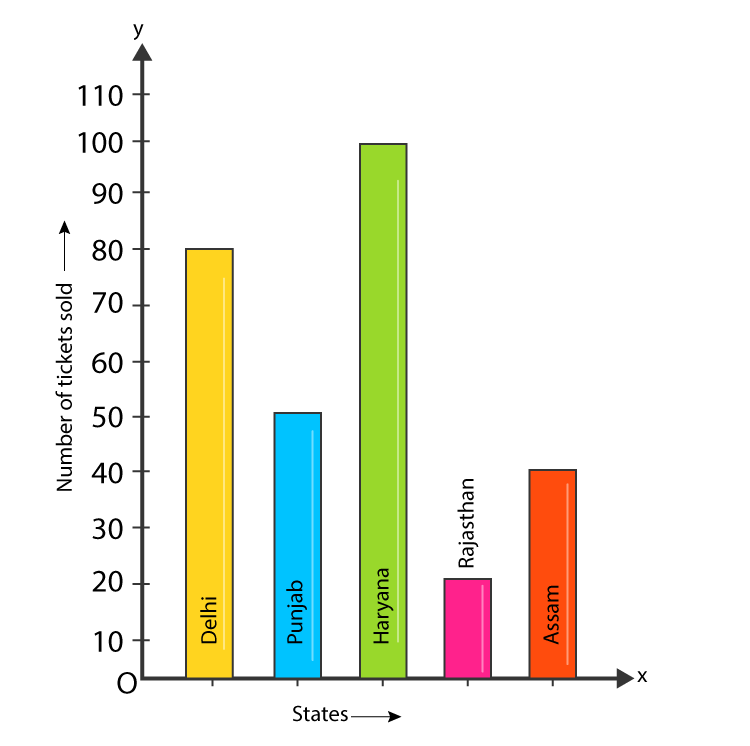Solution:

(i) Bar graph represents the number of tickets of different state lotteries sold by an agent on a day.

(ii) Number of tickets of Assam State Lottery were sold by the agent = 40.

(iii) Maximum numbers of tickets were sold is 100, in the state Haryana.

(iv) Maximum number of tickets were sold is 100, in the state Haryana. Minimum number of tickets were sold is 20, in the state Rajasthan.

It is clear that 100 are equal to 5 times of 20.

Hence, the statement is false.

(v) The minimum numbers of tickets were sold is 20, in the state Rajasthan.

Question 5: Study the bar graph representing the number of persons in various age groups in a town shown in figure. Observe the bar graph and answer the following questions:

(i) What is the percentage of the youngest age-group persons over those in the oldest age group?

(ii) What is the total population of the town?

(iii) What is the number of persons in the age-group 60-65?

(iv) How many persons are more in the age-group 10-15 than in the age group 30-35?

(v) What is the age-group of exactly 1200 persons living in the town?

(vi) What is the total number of persons living in the town in the age-group 50-55?

(vii) What is the total number of persons living in the town in the age-groups 10-15 and 60-65?

(vii) Whether the population in general increases, decreases or remains constant with the increase in the age-group.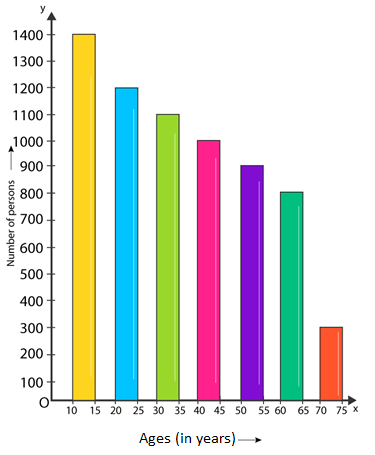Solution:

(i) Youngest age-group is 10-15 years

The number of persons belonging to this group = 1400

The oldest age-group is 70-75 years and

The number of persons belonging to this group = 300

Now,

The percentage of youngest age-group persons over those in the oldest group is as below:

1400/300 × 100 = 1400/3

(ii) Population of the town = 300 + 800 + 900 + 1000 + 1100 + 1200 + 1400 = 6700

(iii) Number of persons in the age group 60 – 65 = 800.

(iv) Number of persons in the age group 10 – 15 = 1400

Number of persons in the age group 30-35 = 1100.

Hence the number of more persons in the age group 10 – 15 than the group 30-35 is 1400 — 1100 = 300.

(v) Age group of 1200 persons living in the town is 20 – 25.

(vi) Total number of persons living in the town in the age-group 50 – 55 is 900.

(vii) Total number of persons living in the town in the age-groups 10 -15 and 60 – 65 is 1400 + 800 = 2200.

(viii) We have observed that, the height of the bars decreases as the age-group increases. Hence, the population decreases with the increases in the age-group.

## RD Sharma Solutions for Class 9 Maths Chapter 23  Graphical Representation of Statistical Data Exercise 23.1

RD Sharma Solutions Class 9 Maths Chapter 23 Graphical Representation of Statistical Data Exercise 23.1 is based on following topics:

• Bar Graph
• Reading and interpretation of bar graphs